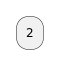# Could functions and procedures, in the preprocessing function call themselves (i.e. be recursive)?

I'm elaborating on the processing shown in the documentation here: https://plantuml.com/preprocessing-json#fe16b3bc321e147d (Self-descriptive example, in the JSON-preprocessing section) and I wondered if it would be possible to allow functions to call themselves? I would allow something like the following to work better:In the working code above, the function: \$sys_diagram calls a copy/pasted (with a different name: \$sys_diagram_sub) function, that in turn calls the original function (\$sys_diagram) which therefor can loop as many times as required to build the desired diagram depth - i didn't expect that to work, I'll be honest, but the system errors when you simply call the \$sys_diagram function from within itself. It would be nice to remove the duplication.

commented Mar 28 by (1,040 points)

answered Mar 28 by (8,280 points)

As you've subsequently discovered, it is fine for a preprocessor procedure/function to call recursively (up to a hardcoded limit)

@startuml
!procedure \$more_rectangles(\$level)
!if \$level > 0
rectangle RECT_##\$level
\$more_rectangles(\$level - 1)
!endif
!endprocedure

\$more_rectangles(10)
@enduml

commented Mar 28 by (45,820 points)

Hello all,

For procedure (with your example) that seems OK:But with function that seems KO:

```@startuml
!function \$f(\$n)
!if (\$n == 0)
!return 0
!elseif (\$n == 1)
!return 1
!else
!return \$f(\$n - 1) + \$f(\$n - 2)
!endif
!end function

:\$f(3);
@enduml```

Do I have something wrong?
It is a defect?

Regards.

commented Mar 30 by (8,280 points)

Interesting.  There is definitely a defect there, but getting to the exact nature of it is tricky.  I've had a go with some-recursive-functions-dont-work-as-expected
The way to get your function above working is:

```@startuml
!function \$f(\$n)
!if (\$n == 0)
!return 0
!elseif (\$n == 1)
!return 1
!else
!return %intval(\$f(\$n - 1)) + %intval(\$f(\$n - 2))
!endif
!end function

:\$f(3);
@enduml
```